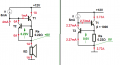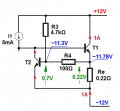# Power amplifier output stage (current limitation, power limitation question)

#### Kartschu Weger

Joined Jan 20, 2019
3
Hello,

I'm designing a power amplifier and the following circuit is the output stage. My output stage consists of two complementary transistors for both, positive and negative waves but for better understanding, I will only show the one which handles the positive waves.Vcc = 12V
Output current (max) = 1.1A
Output voltage (max) = ~ 8V
IN = Sinus with ~8V amplitude and an extremely low (~250uA) current as the discrete Darlington needs a low input current.

As far as I know, R1, R3 & R4, D1 & Q3 are for current & power limitation. How does that work and how can I calculate these three resistors?

Thank you! I would be very happy for every help I can get.

#### MrChips

Joined Oct 2, 2009
24,621
You are not designing a power amplifier. You are copying someone's theoretical design.
We have no idea how to calculate those values.

Why not stick with a more conventional design?

Go to Rod Elliot's site for some design ideas:

http://sound.whsites.net/projects-0.htm#pwr

•Kartschu Weger

#### bertus

Joined Apr 5, 2008
21,680
Hello,

Have a look at the tattached PDF.
The chapters 5 and 6 will tell you a lot about the power stages.

Bertus

#### Attachments

• 4.7 MB Views: 2
•Kartschu Weger

#### Jony130

Joined Feb 17, 2009
5,251
First I should ask if you understand how this simple current protection circuit work?Where I simplified the circuit and T1 represent your Darlington stage with the β = 1000. And T2 and I1 as overcurrent protection.
The driver (VAS - voltage amplifier stage) and the bias stages were not shown.

In normal condition, T2 is OFF the amplifier works as if there was no T2 in a circuit.

To open the T2 transistor the voltage across RE resistor should be around 0.6V. Therefore as soon as the load current increases to the value so that the voltage drop across RE becomes larger than 0.6V the T2 will start to Turn-ON. When T2 turns-ON, starts to steal the base current from T1 (start pulling I1 current away from the T1 base) thereby reducing the collector current of T1. Hence the output current is limited to I_max ≈ 0.7V/Re.
Also notice that the crucial thing for this circuit to work properly is that the driver stage (I1 = 5mA in my diagram) can only provide a limited amount of current. If this condition is not meet the circuit will not work.

Now adding the voltage divider (R3, R4) to the T2 makes that now the IL_max will no longer be fixed ( at Imax = 0.7V/Re) but will vary together with T1 Vce voltage. But the main principle of operation stays the same.

And again the IL_max is the same and equal to Imax ≈ 0.7V/Re ≈ 0.7V/0.22Ω ≈ 3.2A . But if we want to reduce this current at short to Vee (-12V) to let us say 1A. We can achieve this if we use R4 = 100Ω and VR4 = (0.7 V - 1A*0.22Ω) = 0.48V; and R4 resistor current is
IR4 = 0.48V/100Ω = 4.8V and finaly

R3 = (24V - 0.7V)/4.8mA ≈ 4.7kΩLast edited:
•Kartschu Weger